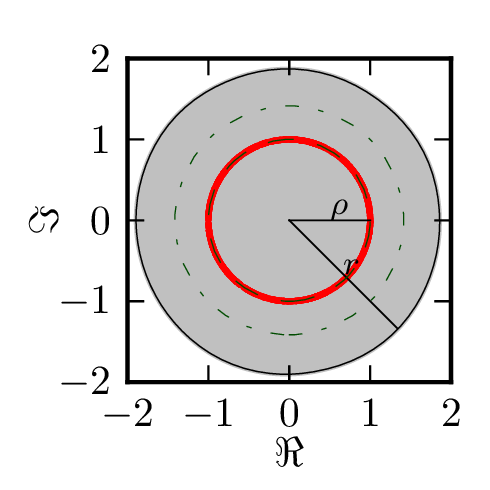# Numerical range of random matrices

The figures below depict numerical range for large random matrices drawn from different ensembles. Gray areas denote numerical ranges and red dots denote spectra of matrices.

Matrices are normalized so that for every matrix $A$ $\Tr(AA^\dagger)=\dim(A)$.

In the figures below

• $r(X)= {\rm max}\{|z|: z \in W(X)\}$ denotes the matrix numerical radius
• $\rho(X)= |\lambda_{\max}|$ denotes the matrix spectral radius,

where $\lambda_{\rm max}$ is the leading eigenvalue of $X$ with the largest modulus.

## Definitions

Complex Ginibre matrices $G_d$ of dimention $d$ with entries $\xi_{ij}$, where $\mathbb{E} |\xi _{ij}|^2 =1/d$. As we mention in the introduction, by the circular law, the spectrum of $G_N$ is asymptotically contained in the unit disk. Note $\|G_d\| _{}^2=d$.

Upper triangular random matrices $T_d$ of dimension $d$ with entries $T_{ij}=\xi_{ij}$ for $i <j$ and $T_{ij}=0$ elsewhere, where $\mathbb{E} |\xi _{ij}|^2 =2/(d-1)$. Clearly, all eigenvalues of $T_N$ equal to zero. Note $\|T_d\| _{}^2=d$.

Diagonalized Ginibre matrices, $D_d = Z G_d Z^{-1}$ of dimension $d$, so that $D_{kl}=\lambda_k \delta_{kl}$ where $\lambda_k$, $k=1,\dots, d$, denote complex eigenvalues of $G_d$. In order to ensure the uniqueness of the probability distribution on diagonal matrices, we assume that it is invariant under conjugation by permutations. Note that integrating over the Girko circular law one gets the average squared eigenvalue of the complex Ginibre matrix, $||2=*{0}^1 2x^3 dx=1/2$. Thus,$\|D_d\| *{}2=d/2$.

Diagonal unitary matrices $U_d$ of order $d$ with entries $U_{kl}=\exp(i \phi_k) \delta_{kl}$, where $\phi_k$ are independent uniformly distributed on $[0, 2 \pi)$ real random variables.

Examples:

$G_4 = \begin{pmatrix} \xi & \xi & \xi & \xi \\ \xi & \xi & \xi & \xi \\ \xi & \xi & \xi & \xi \\ \xi & \xi & \xi & \xi \\ \end{pmatrix}, \;\; T_4 = \begin{pmatrix} 0 & \xi & \xi & \xi \\ 0 & 0 & \xi & \xi \\ 0 & 0 & 0 & \xi \\ 0 & 0 & 0 & 0 \\ \end{pmatrix}, \;\; D_4 = \begin{pmatrix} \lambda_1 & 0 & 0 & 0 \\ 0 & \lambda_2 & 0 & 0 \\ 0 & 0 & \lambda_3 & 0 \\ 0 & 0 & 0 & \lambda_4 \\ \end{pmatrix}.$

### Matrices drawn from Ginibre ensemble

A matrix $G_{10}$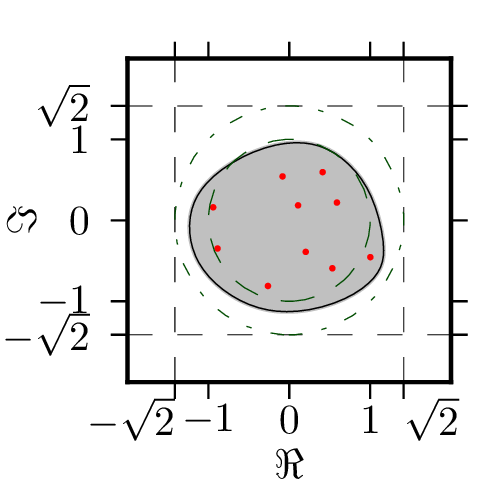A matrix $G_{100}$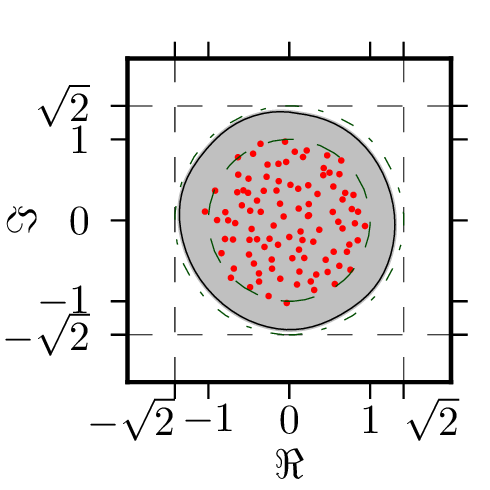A matrix $G_{1000}$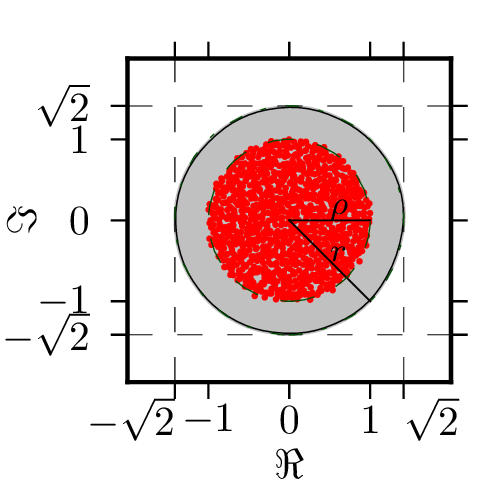## Diagonal matrices

A matrix $D_{10}$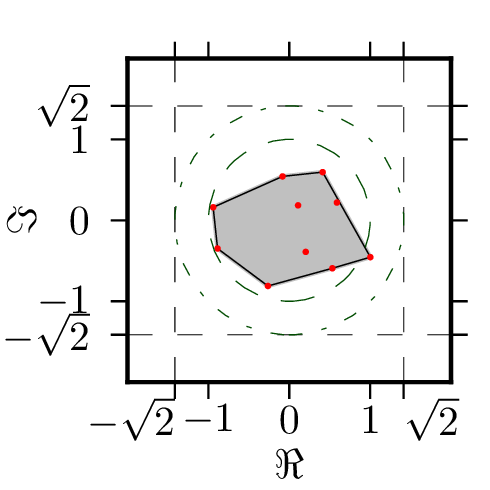A matrix $D_{100}$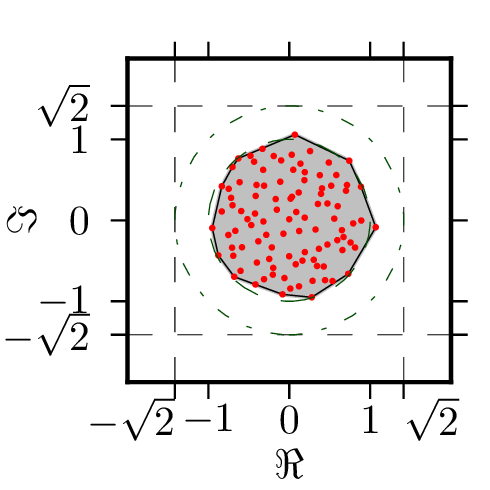A matrix $D_{1000}$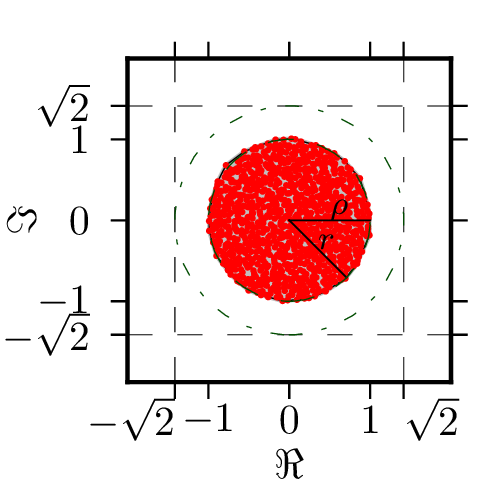### Strictly upper triangular matrices

A matrix $T_{10}$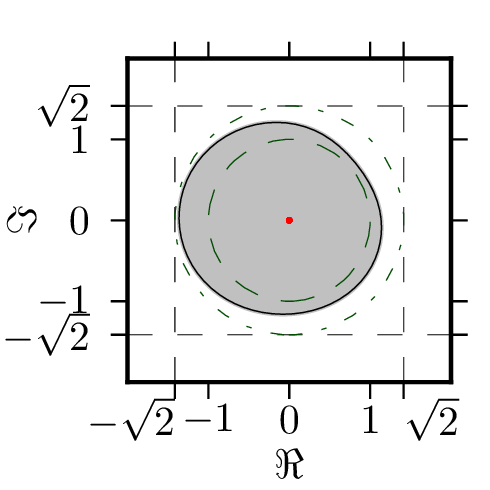A matrix $T_{100}$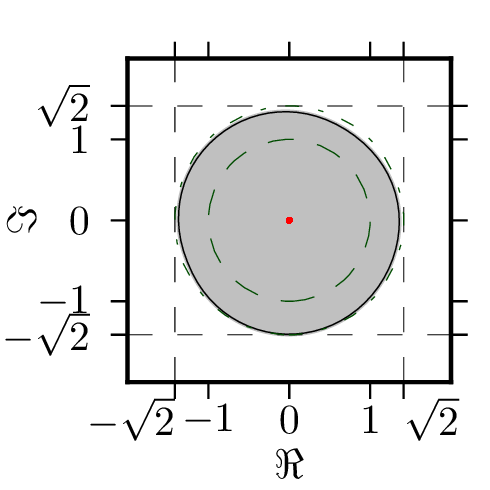A matrix $T_{1000}$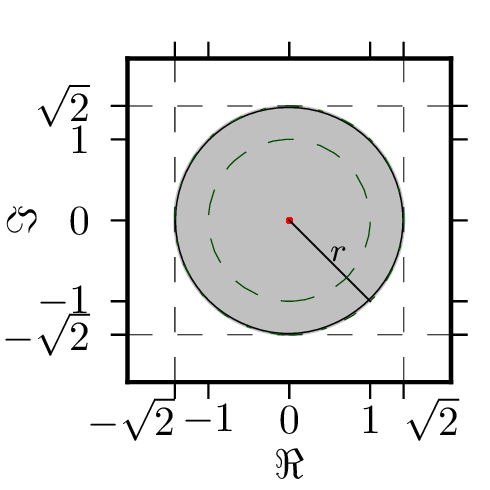### Combinations

A matrix $D_{1000}+1/\sqrt{2}T_{1000}$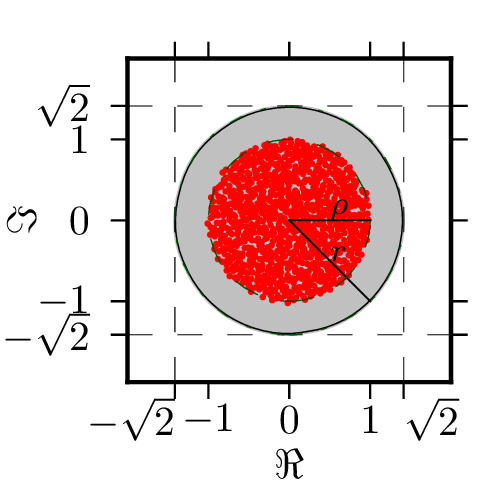A matrix $U_{1000}+T_{1000}$